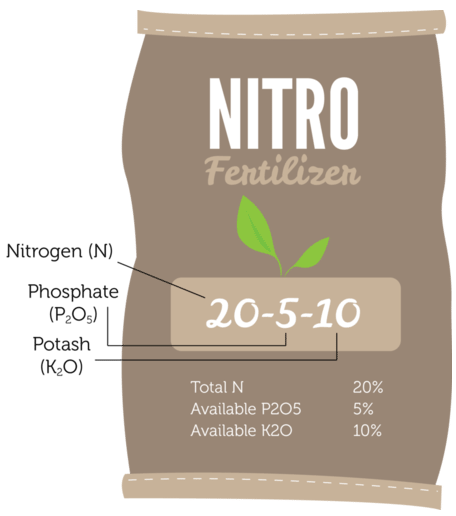# 6.9: Hydrogen and Alkali Metals

You probably think of water as a substance that can put out fires. But some elements are so reactive that they burn in water. In fact, they virtually explode in water. An example is shown in the photo below. About 3 pounds of sodium were added to water, and the result was an explosive reaction. Why is sodium such a reactive element? In this section, you will find out.Figure $$\PageIndex{1}$$: Sodium reacting with water.

## The First Group

Sodium $$\left( \ce{Na} \right)$$ is an element in group 1 of the periodic table of the elements. This group (column) of the table is shown below. It includes the nonmetal hydrogen $$\left( \ce{H} \right)$$ and six metals called alkali metals. Elements in the same group of the periodic table have the same number of valence electrons. These are the electrons in the outer energy level of the atoms that can be involved in chemical reactions. Valence electrons determine many of the properties of an element, so elements in the same group have similar properties. All the elements in group 1 have just one valence electron. This makes them very reactive.Figure $$\PageIndex{2}$$: Group 1 of the periodic table.

## Reactivity of Group 1 Elements

Hydrogen is a very reactive gas, and the alkali metals are even more reactive. In fact, they are the most reactive metals and, along with the elements in group 17, are the most reactive of all elements. The reactivity of alkali metals increases from the top to the bottom of the group, so lithium $$\left( \ce{Li} \right)$$ is the least reactive alkali metal and francium $$\left( \ce{Fr} \right)$$ is the most reactive. Because alkali metals are so reactive, they are found in nature only in combination with other elements. They often combine with group 17 elements, which are very "eager" to gain an electron.

## Other Properties of Alkali Metals

Besides being very reactive, alkali metals share a number of other properties:

• Alkali metals are all solids at room temperature.
• Alkali metals are low in density, and some of them float on water.
• Alkali metals are relatively soft. Some are even soft enough to cut with a knife, like the sodium pictured in the figure below.Figure $$\PageIndex{3}$$: Elemental sodium is soft enough to cut with a knife.

## A Closer Look

Although all group 1 elements share certain properties, such as being very reactive, they are not alike in every way. Three different group 1 elements are described in more detail below. Notice the ways in which they differ from one another.Figure $$\PageIndex{4}$$: Hydrogen has the smallest, lightest atoms of all elements. Pure hydrogen is a colorless, odorless, tasteless gas that is nontoxic but highly flammable. Hydrogen gas exists mainly as diatomic ("two-atom") molecules $$\left( \ce{H_2} \right)$$, as shown above. Hydrogen is the most abundant element in the universe and the third most abundant element on Earth, and occurs mainly in compounds such as water.Figure $$\PageIndex{5}$$: Potassium is a soft, silvery metal that ignites explosively in water. It easily loses its one valence electron to form positive potassium ions $$\left( \ce{K^+} \right)$$, which are needed by all living cells. Potassium is so important for plants that it is found in almost all fertilizers, like the one shown here. Potassium is abundant in Earth's crust in minerals such as feldspar.Figure $$\PageIndex{6}$$: Francium has one of the largest, heaviest atoms of all elements. Its one valence electron is far removed from the nucleus, as you can see in the atomic model above, so it is easily removed from the atom. Francium is radioactive and quickly decays to form other elements, such as radium. This is why francium is extremely rare in nature. Less than an ounce of francium is present on Earth at any given time.

## Summary

• Group 1 of the periodic table includes hydrogen and the alkali metals.
• Because they have just one valence electron, group 1 elements are very reactive. As a result, they are found in nature only in combination with other elements.
• Alkali metals are all solids at room temperature. They are relatively soft and low in density.
• From the top to the bottom of group 1, the elements have heavier, more reactive atoms.

## Explore More

At the following URL, click on "Group 1 data and graphs". Use the data in the group 1 table to fill in the blanks below with either increases or decreases.

From the top to the bottom of group 1, properties of the alkali metals change as follows:

1. Melting point _________.

2. Boiling point _________.

3. Density _________.